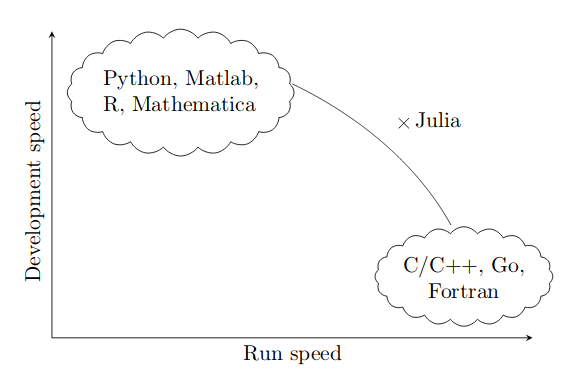Julia is a free language that is easy to use and is specifically suitable for machine learning, data science, and scientific computing. It was first public in 2012 and its first stable version (v1.0) was released in 2018. The language has been inspired by many of good design features from other languages and uses new technologies in compilation and optimization. It runs as fast as C/C++ and Fortran, and at the same time, is easy to write, similar to Python, Matlab, and R.

A proof that Julia solves the two language problem is that most of the of language itself and standard libraries are written in Julia. It makes learning and extending the language easy.

We want a language that’s open source, with a liberal license. We want the speed of C with the dynamism of Ruby. We want a language that’s homoiconic, with true macros like Lisp, but with obvious, familiar mathematical notation like Matlab. We want something as usable for general programming as Python, as easy for statistics as R, as natural for string processing as Perl, as powerful for linear algebra as Matlab, as good at gluing programs together as the shell. Something that is dirt simple to learn, yet keeps the most serious hackers happy. We want it interactive and we want it compiled. (Did we mention it should be as fast as C?)

## Why is it worth to learn Julia

### Speed and ease of codingFig. 1. Julia has the best of both worlds. Image from 'Statistics with Julia', Klok and Nazarathy.

When trying to quantify speed, the answer is not simple. On the one hand, speed can be quantified in terms of how fast a piece of computer code runs, namely runtime speed. On the other hand, speed can be quantified in terms of how fast it takes to code, debug and re-factor computer code, namely development speed. Within the realm of scientific computing and statistical computing, compiled low-level languages such as Fortran, C/C++ and the like generally yield fast runtime performance, however require more care in creation of the code. Hence they are generally fast in terms of runtime, yet slow in terms of development time. On the opposite side of the spectrum are mathematically specialized languages such as Mathematica, R, Matlab as well as Python. These typically allow for more flexibility when creating code, hence generally yield quicker development times. However, run times are typically significantly slower than what can be achieved with a low-level language. In fact, many of the efficient statistical and scientific computing packages incorporated in these languages are written in low-level languages, such as Fortran or C/C++,which allows for faster runtimes when applied as closed modules.

While speed (both development and runtime) is hard to fully and fairly quantify, Figure 1 illustrates a schematic view showing general speed trade-offs between languages. As is postulated by this figure, there is a type of a Pareto optimal frontier ranging from the C language on on end to the R language on the other. The location of each language on this figure cannot be determined exactly. However, few would disagree that “R is generally faster to code than C” and “C generally runs faster than R”

### Ecosystem

Julia has a fastly growing ecosystem of high-quality libraries. The following are some notable examples:

• DataFrames: A library for working with tabular data. It provides functionalities similar to R’s data frame and Python’s Pandas. See DataFrames for more details.
• Flux: Flux is a library for machine learning. It comes “batteries-included” with many useful tools built in, but also lets you use the full power of the Julia language where you need it.
• Scikitlearn: ScikitLearn.jl implements the popular Python machine learning library “scikit-learn” interface and algorithms in Julia.

### Language interoperability

Although Julia already has many useful libraries, but older languages still have many more. Fortunately, using Julia, you will not miss those libraries. Using C and Python libraries, especially, are pretty straight forward. You can interact with the following languages.

• Python
• C
• C++
• R
• Matlab

This is an example of using some Python libraries with `PyCall`:

``````using Pycall
math = pyimport("math")  # imports the math library from Python
math.sin(math.pi / 4) # use any function from that library
``````

### Language design

• Easy syntax
• Multiple dispatch

### Julia for machine learning

• Syntax looks like pseudo-code
• Large standard library
• Good performance
• Parallelism
• Open source

### Parallel computing

Parallel computing is a way of dealing with data in parallel ways. It uses multiple CPUs or CPU cores to perform computation. This will be very important when dealing with large data and intensive computations. Julia includes builtin support for all types of parallel computing (see the Parallel Computing section).

Tags: